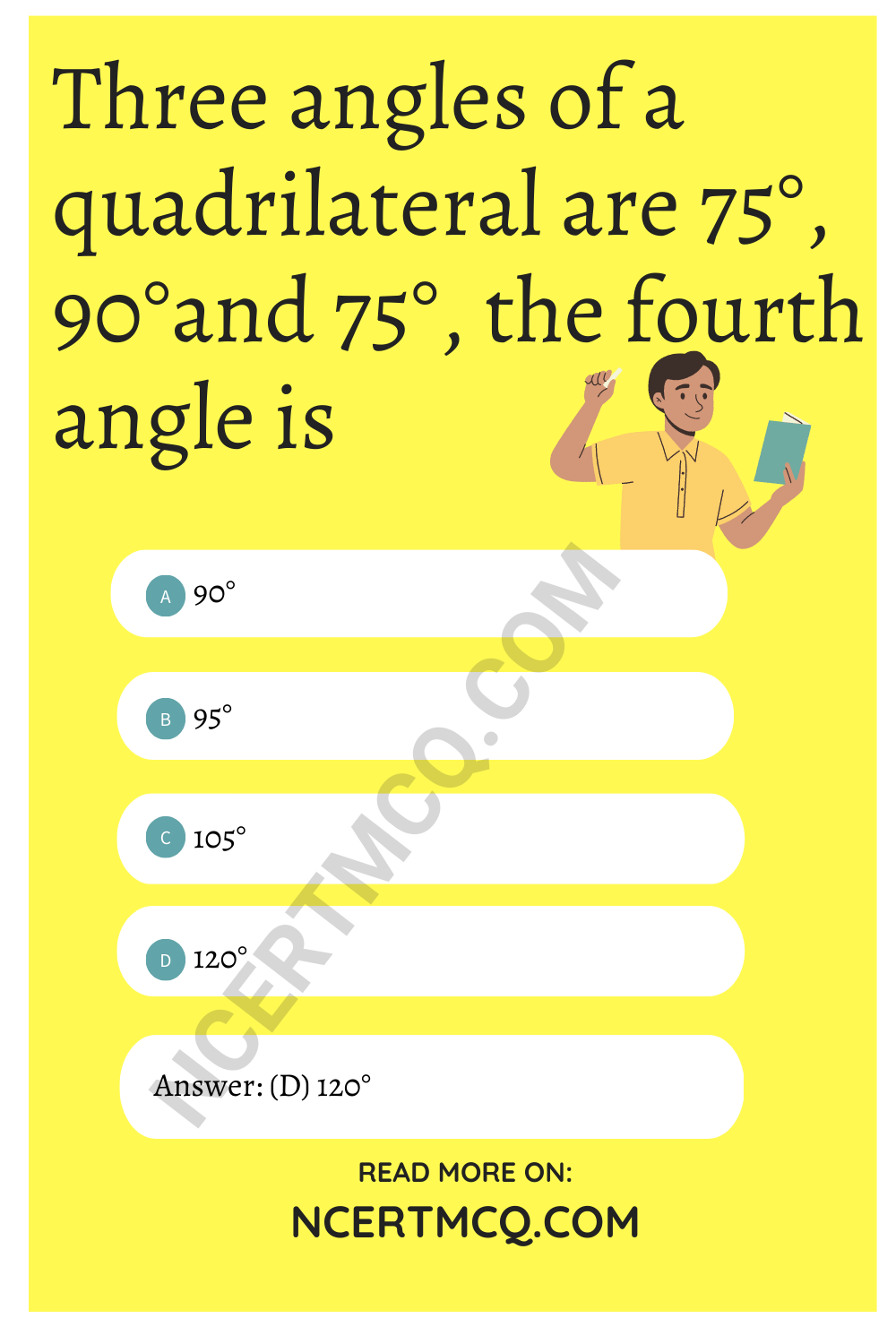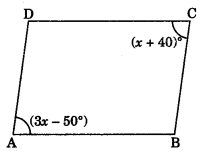Check the below Online Education NCERT MCQ Questions for Class 9 Maths Chapter 8 Quadrilaterals with Answers Pdf free download. MCQ Questions for Class 9 Maths with Answers were prepared based on the latest exam pattern. We have provided Quadrilaterals Class 10 Maths MCQs Questions with Answers to help students understand the concept very well. https://ncertmcq.com/mcq-questions-for-class-9-maths-with-answers/

Students can also refer to NCERT Solutions for Class 9 Maths Chapter 8 Quadrilaterals for better exam preparation and score more marks.

Quadrilateral Class 9 MCQ Question 1.
Three angles of a quadrilateral are 75°, 90°and 75°, the fourth angle is
(a) 90°
(b) 95°
(c) 105°
(d) 120°Quadrilateral MCQ Class 9 Question 2.
A diagonal of a rectangle is inclined to one side of the rectangle at 25°. The acute angle between the diagonals is
(a) 55°
(b) 50°
(c) 40°
(d) 25°

ABCD is a rhombus such that ∠ACB = 40°, then ∠ADB is
(a) 40°
(b) 45°
(c) 50°
(d) 60°

Quadrilaterals Class 9 MCQ Question 4.
If angles A, B, C and D of a quadrilateral ABCD, taken in order, are in the ratio 3 : 7 : 6 : 4, then ABCD is a
(a) rhombus
(b) parallelogram
(c) trapezium
(d) kite.

MCQ On Quadrilaterals Class 9 Question 5.
The diagonals AC and BD of a || gm ABCD intersect each other at the point O. If ∠DAC = 32° and ∠AOB = 70°, then ∠DBC is equal to
(a) 24°
(b) 86°
(c) 38°
(d) 32°

Class 9 Quadrilaterals MCQ Question 6.
ABCD is a rhombus such that ∠ABC = 40°, then ∠ADC is equal to
(a) 40°
(b) 45°
(c) 50°
(d) 20°

Class 9 Maths Chapter 8 MCQ With Answers Question 7.
In the following figure, ABCD and AEFG are two parallelograms. If ∠C = 60°, then ∠GFE is(a) 30°
(b) 60°
(c) 90°
(d) 120°

MCQ Quadrilaterals Class 9 Question 8.
The bisectors of any two adjacent angles of a || gm intersect at
(a) 30°
(b) 45°
(c) 60°
(d) 90°

MCQ Of Quadrilaterals Class 9 Question 9.
If one angle of a parallelogram is 24° less than twice the smallest angle, then the measure of the largest angle of a parallelogram is
(a) 176°
(b) 68°
(c) 112°
(d) 102°

Class 9 Maths Chapter 8 MCQ Question 10.
If the diagonal of a rhombus are 18 cm and 24 cm respectively, then its side is equal to
(a) 16 cm
(b) 15 cm
(c) 20 cm
(d) 17 cmClass 9 Maths Quadrilaterals MCQ Question 11.
In the given figure, ABCD is a parallelogram. Find the value of x.(a) 25°
(b) 60°
(c) 75°
(d) 45°